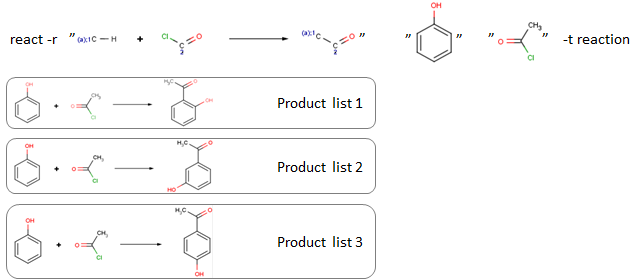# Product list

If there are more reaction centers in a reactant, reaction could lead to diverse products (e.g., isomers). Product list is the output of a reaction on one reaction center of the reactant. REVIEW THIS PAGE

`react -r "[c:1][H].[Cl][C:2]=[O]>>[c:1][C:2]=[O]" "OC1=CC=CC=C1" "CC(Cl)=O" -t reactionOC1=CC=CC=C1.CC(Cl)=O>>CC(=O)C1=C(O)C=CC=C1OC1=CC=CC=C1.CC(Cl)=O>>CC(=O)C1=CC=CC(O)=C1OC1=CC=CC=C1.CC(Cl)=O>>CC(=O)C1=CC=C(O)C=C1`